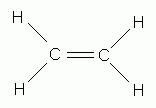What are the steps associated with the process of constructing a hybrid orbital diagram?

Dec 13, 2014

Here are the steps for constructing a hybrid orbital diagram for ethylene.

Step 1. Draw the Lewis structure for the molecule.Step 2. Use VSEPR theory to classify and determine the geometry around each central atom.Each carbon atom is an AX₃ system, so the geometry is trigonal planar.

Step 3. Determine the hybridization that corresponds to this geometry.Trigonal planar geometry corresponds to sp² hybridization.

Step 4. Draw the two carbon atoms side-by-side with their orbitals,Step 5. Bring the C atoms and H atoms together to show the overlap of orbitals forming the σ and π bonds.Here’s a video on how to draw a hybrid orbital diagram.